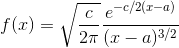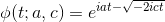# Lévy Distribution: Definition, PDF, Examples

Share on

Probability Distributions

The Lévy Distribution is a probability distribution that is both continuous—for non-negative random variables—and stable.

To be stable means that if you sum up random variables x and y in a distribution, their sum (i.e. x + y) is a random variable from that same distribution.

Another important property of the Lévy distribution is that its probability density function (PDF) can be expressed analytically. In other words, we can write equations for them and analyze their pdfs mathematically; This makes it much easier to work with. The only other stable distributions that can be expressed analytically are the normal distribution and the Cauchy distribution.

# Probability Density Function for the Lévy distribution

The PDF is:Where:
c = the scale parameter; c > 0.
a = the location parameter (this parameter is sometimes given the variable μ.); x ≥ a.

# The Characteristic Function for the Lévy Distribution

The characteristic function for this distribution is just:Here a and c are the scale parameters and location parameters, with the same boundaries as above.

# Using the Lévy Distribution

Finance engineers often choose the Lévy Distribution to model price changes in markets. The ‘fat tail‘ or slow fall off that this distribution models is a good match for what happens after prices change.

But that is one of many possible applications, both in social engineering and physical sciences. Another application: when a photon travels in a turbid medium, the length of its path follows the Lévy distribution. The frequency of geomagnetic reversals (“pole shifts”) appears to follow this distribution as well. And when a particle is in Brownian motion, the time it will take to hit a single point α can be modeled by the Levy distribution, where c, our scale parameter, is just α2

## References

Carbone, V.et. al (2006). “Clustering of Polarity Reversals of the Geomagnetic Field”. Physical Review Letters. 96 (12): 128501.
Dumé, Belle (March 21, 2006). “Geomagnetic flip may not be random after all“. physicsworld.com. Retrieved December 27, 2017.
Lucas, A. (n.d.) Random Variables: Levy Distributions, Stanford University. Retrieved December 18, 2017 from:
https://web.stanford.edu/~ajlucas/Levy%20Distributions.pdf

CITE THIS AS:
Stephanie Glen. "Lévy Distribution: Definition, PDF, Examples" From StatisticsHowTo.com: Elementary Statistics for the rest of us! https://www.statisticshowto.com/levy-distribution/
---------------------------------------------------------------------------Need help with a homework or test question? With Chegg Study, you can get step-by-step solutions to your questions from an expert in the field. Your first 30 minutes with a Chegg tutor is free!